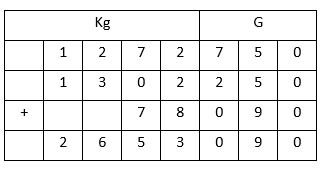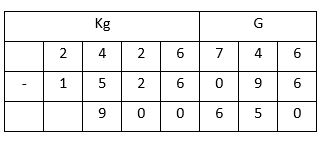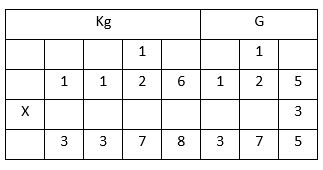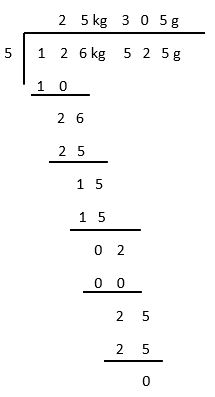## LetsPlayMaths.Com

WELCOME TO THE WORLD OF MATHEMATICS

# Class 4 Weight

Measurement of Weight

Addition of Weight

Subtraction of Weight

Multiplication of Weight

Division of Weight

Weight / Mass Test

Weight / Mass Worksheet

Answer Sheet

## Measurement of Weight

We have learnt in class III that the unit of weight is kilogram. In short form we write it as kg. Bigger things are weighs in kilogram and the smaller things are weighs in grams. Let’s see which all things are weighs in kilograms and which are in grams.

Kilograms

1. One bag of cement

2. One bag of rice

3. One packet of sugar

4. One packet of salt

Grams

1. Gold and silver in grams

1. Curry powder in grams

1. Letters in grams

As we know, 1000 grams = 1 kg## Addition of Weight

We have learnt the addition of weights in class III. Here, we will use the same methods to add the bigger quantities. We can add kilograms and grams like normal numbers, but grams should be written as three digits numbers. For example, 45 grams should be written as 045 grams and 5 grams should be written as 005 grams.

Let’s have a look at some examples.

Example 1. Add 432 kg 175 g and 126 kg 57 g.

Solution.So, the answer is 558 kg 232 grams.

Example 2. Add 1272 kg 750 grams, 1302 kg 250 grams and 78 kg 90 grams.

Solution.So, the answer is 2653 kg 90 grams.

## Subtraction of Weight

We can subtract kilograms and grams as normal subtraction. But, grams should be written as three-digit numbers. Let’s have a look at some examples.

Example 1. Subtract 1526 kg 96 g from 2426 kg 746 g.

Solution. Write 2426 kg 746 g and 1526 kg 96 g in tabular format.So, the answer is 900 kg 650 grams.

## Multiplication of Weight

We can multiply kilograms and grams as normal multiplication. But, grams should be written as three-digit numbers. Let’s have a look at some examples.

Example 1. Multiply 1126 kg 125 g by 3.

Solution. Write 1126 kg 125 g and 3 in tabular format as shown below.So, the answer is 3378 kg 375 g.

## Division of Weight

Division of kilograms and grams can be done with normal division. In this method, grams must be written in 3-digit format.

Example 1. Divide 126 kg 525 g by 5.

Solution.So, the answer is 25 kg 305 g

Weight Test - 1

Weight Test - 2

## Class-4 Weight/Mass Worksheet

Weight Worksheet - 1

Weight Worksheet - 2

## Answer Sheet

Weight-AnswerDownload the pdf

Copyright © 2021 LetsPlayMaths.com. All Rights Reserved.